# Resources tagged with: Place value

Filter by: Content type:
Age range:
Challenge level:

### There are 91 results

Broad Topics > The Number System and Place Value > Place value### One Million to Seven

##### Age 7 to 11 Challenge Level:

Start by putting one million (1 000 000) into the display of your calculator. Can you reduce this to 7 using just the 7 key and add, subtract, multiply, divide and equals as many times as you like?### Oddly

##### Age 7 to 11 Challenge Level:

Find the sum of all three-digit numbers each of whose digits is odd.### Being Resilient - Primary Number

##### Age 5 to 11 Challenge Level:

Number problems at primary level that may require resilience.##### Age 5 to 11 Challenge Level:

Try out this number trick. What happens with different starting numbers? What do you notice?### Being Collaborative - Primary Number

##### Age 5 to 11 Challenge Level:

Number problems at primary level to work on with others.### Being Resourceful - Primary Number

##### Age 5 to 11 Challenge Level:

Number problems at primary level that require careful consideration.### Six Is the Sum

##### Age 7 to 11 Challenge Level:

What do the digits in the number fifteen add up to? How many other numbers have digits with the same total but no zeros?### Spell by Numbers

##### Age 7 to 11 Challenge Level:

Can you substitute numbers for the letters in these sums?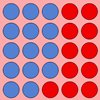### Place Value as a Building Block for Developing Fluency in the Calculation Process

##### Age 5 to 11

This article for primary teachers encourages exploration of two fundamental ideas, exchange and 'unitising', which will help children become more fluent when calculating.### Napier's Bones

##### Age 7 to 11 Challenge Level:

The Scot, John Napier, invented these strips about 400 years ago to help calculate multiplication and division. Can you work out how to use Napier's bones to find the answer to these multiplications?### ABC

##### Age 7 to 11 Challenge Level:

In the multiplication calculation, some of the digits have been replaced by letters and others by asterisks. Can you reconstruct the original multiplication?### Alien Counting

##### Age 7 to 11 Challenge Level:

Investigate the different ways these aliens count in this challenge. You could start by thinking about how each of them would write our number 7.### Journeys on the Gattegno Tens Chart

##### Age 5 to 11

Alf describes how the Gattegno chart helped a class of 7-9 year olds gain an awareness of place value and of the inverse relationship between multiplication and division.### Multiply Multiples 3

##### Age 7 to 11 Challenge Level:

Have a go at balancing this equation. Can you find different ways of doing it?### Multiply Multiples 1

##### Age 7 to 11 Challenge Level:

Can you complete this calculation by filling in the missing numbers? In how many different ways can you do it?### All the Digits

##### Age 7 to 11 Challenge Level:

This multiplication uses each of the digits 0 - 9 once and once only. Using the information given, can you replace the stars in the calculation with figures?### Activities on the Gattegno Chart

##### Age 5 to 11

In this article, Alf outlines six activities using the Gattegno chart, which help to develop understanding of place value, multiplication and division.### Trebling

##### Age 7 to 11 Challenge Level:

Can you replace the letters with numbers? Is there only one solution in each case?### Song Book

##### Age 7 to 11 Challenge Level:

A school song book contains 700 songs. The numbers of the songs are displayed by combining special small single-digit cards. What is the minimum number of small cards that is needed?### Arrange the Digits

##### Age 11 to 14 Challenge Level:

Can you arrange the digits 1,2,3,4,5,6,7,8,9 into three 3-digit numbers such that their total is close to 1500?### Number Detective

##### Age 5 to 11 Challenge Level:

Follow the clues to find the mystery number.### Multiply Multiples 2

##### Age 7 to 11 Challenge Level:

Can you work out some different ways to balance this equation?##### Age 11 to 14 Challenge Level:

By selecting digits for an addition grid, what targets can you make?##### Age 5 to 11 Challenge Level:

Who said that adding couldn't be fun?### Coded Hundred Square

##### Age 7 to 11 Challenge Level:

This 100 square jigsaw is written in code. It starts with 1 and ends with 100. Can you build it up?### Diagonal Sums

##### Age 7 to 14 Challenge Level:

In this 100 square, look at the green square which contains the numbers 2, 3, 12 and 13. What is the sum of the numbers that are diagonally opposite each other? What do you notice?### The Thousands Game

##### Age 7 to 11 Challenge Level:

Each child in Class 3 took four numbers out of the bag. Who had made the highest even number?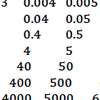### How Can I Support the Development of Early Number Sense and Place Value?

##### Age 3 to 11

This article for primary teachers expands on the key ideas which underpin early number sense and place value, and suggests activities to support learners as they get to grips with these ideas.##### Age 11 to 14 Challenge Level:

Visitors to Earth from the distant planet of Zub-Zorna were amazed when they found out that when the digits in this multiplication were reversed, the answer was the same! Find a way to explain. . . .### Round the Three Dice

##### Age 7 to 11 Challenge Level:

What happens when you round these three-digit numbers to the nearest 100?### Football Sum

##### Age 11 to 14 Challenge Level:

Find the values of the nine letters in the sum: FOOT + BALL = GAME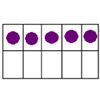### Place Value: the Ten-ness of Ten

##### Age 3 to 11

This article develops the idea of 'ten-ness' as an important element of place value.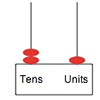### Position

##### Age 5 to 11

One of the key ideas associated with place value is that the position of a digit affects its value. These activities support children in understanding this idea.### Amount

##### Age 5 to 11

These tasks will help learners develop their understanding of place value, particularly giving them opportunities to express numbers as amounts.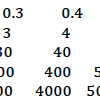### Number Sense and Place Value

##### Age 5 to 11

This feature aims to support you in developing children's early number sense and understanding of place value.### More Upper Primary Activities on Number Sense and Place Value

##### Age 7 to 11

More upper primary number sense and place value tasks.### Nice or Nasty for Two

##### Age 7 to 14 Challenge Level:

Some Games That May Be Nice or Nasty for an adult and child. Use your knowledge of place value to beat your opponent.##### Age 11 to 14 Challenge Level:

What happens when you add a three digit number to its reverse?### Nice or Nasty

##### Age 7 to 14 Challenge Level:

There are nasty versions of this dice game but we'll start with the nice ones...### Subtraction Surprise

##### Age 7 to 14 Challenge Level:

Try out some calculations. Are you surprised by the results?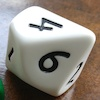### Less Is More

##### Age 5 to 11 Challenge Level:

Use your knowledge of place value to try to win this game. How will you maximise your score?### Which Is Quicker?

##### Age 7 to 11 Challenge Level:

Which is quicker, counting up to 30 in ones or counting up to 300 in tens? Why?### What Do You Need?

##### Age 7 to 11 Challenge Level:

Four of these clues are needed to find the chosen number on this grid and four are true but do nothing to help in finding the number. Can you sort out the clues and find the number?### Cayley

##### Age 11 to 14 Challenge Level:

The letters in the following addition sum represent the digits 1 ... 9. If A=3 and D=2, what number is represented by "CAYLEY"?##### Age 11 to 14 Challenge Level:

Replace the letters with numbers to make the addition work out correctly. R E A D + T H I S = P A G E### Eleven

##### Age 11 to 14 Challenge Level:

Replace each letter with a digit to make this addition correct.### Two and Two

##### Age 11 to 16 Challenge Level:

How many solutions can you find to this sum? Each of the different letters stands for a different number.### Tis Unique

##### Age 11 to 14 Challenge Level:

This addition sum uses all ten digits 0, 1, 2...9 exactly once. Find the sum and show that the one you give is the only possibility.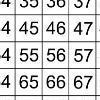### That Number Square!

##### Age 5 to 11 Challenge Level:

Exploring the structure of a number square: how quickly can you put the number tiles in the right place on the grid?### Alphabet Soup

##### Age 11 to 14 Challenge Level:

This challenge is to make up YOUR OWN alphanumeric. Each letter represents a digit and where the same letter appears more than once it must represent the same digit each time.